# End correction of a closed pipe

• Zoro
According to wiki, end correction is:the adjustment of the end of a resonant pipe to compensate for changes in its length, often due to changes in pipe size or age.

## Homework Statement

Is there a scientiically approved method for end correction of a closed pipe.

## The Attempt at a Solution

The formula used is Delta L = (3d) where d = diameter in cm.

Zoro said:
Is there a scientiically approved method for end correction of a closed pipe.

i think there are theoretical analysis of the correction by Rayleigh and others and one will have to go through their work...as people who are manufacturing the organ pipes do take care for the exactness of the frequencies to be excited by the pipes

for example one can see;
Lord Rayleigh, Theory of Sound, Vol II, §314 (Dover, NY, 1945  ) p.201 ff.
http://www.larrylawson.net/Journal/Pipe1V5N2.pdf

Zoro said:
The formula used is Delta L = (3d) where d = diameter in cm.
Check the correction term ;
perhaps it is 0.3 r or 0.6 D where r and D are radius and diameter of the pipe respectively.

According to wiki there is not:

There is no scientifically proven and accepted value for the end correction of a resonant tube, various values ranging from 0.3r to 0.6r have been suggested from numerous disparate experiments. Lord Rayleigh was the first experimenter to publish a figure, in 1871: it was 0.3r.

Take from that what you will.

drvrm said:
Check the correction term ;
perhaps it is 0.3 r or 0.6 D where r and D are radius and diameter of the pipe respectively.
Did you mean "0.6r or 0.3D"?

haruspex said:
Did you mean "0.6r or 0.3D"?

i might have made an error; pl. see
https://en.wikipedia.org/wiki/End_correction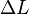is known as end correction, which can be calculated as,

• for a closed pipe (with one opening):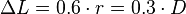,
where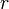is the hydraulic radius of the neck and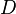is the hydraulic diameter of the neck;

• and for an open pipe (with two openings):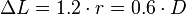.

is it 0.3 d where d is in cm or m